# Volume and Density Definitions and Determination Methods

There are a number of manual and automated methods for determining volume and density. This article, however, focuses on laboratory methods that are most often used in research and quality control applications.

Another large area of application is on-line monitoring in production control.

## The Density Enigma

When first introduced to density, perhaps in grade school, we were taught that it simply is the mass of an object divided by its volume. We thought that was pretty much the whole story, but sooner or later we discovered that this definition was only the beginning. The difficulty in defining density is exemplified by the American Society for Testing and Materials’ book of standard definitions where one finds over forty definitions based on mass per unit volume. The British Standards Institute has narrowed it down to fourteen types of densities.

## Volume Determination and Definition

Determining the mass of an object is rather straightforward; it is the determination of volume that conceals the difficulty. The ‘volume’ of a solid object, whether a single piece or a mass of finely divided powder, is one of those concepts that can’t be bundled up into a single, neat definition.

A layman’s dictionary typically defines volume in vague terms such as ‘the space occupied by an object.’ McGraw-Hill’s Dictionary of Scientific and Technical Terms expands only slightly on that definition, offering “A measure of the size of a body or definite region in three dimensional space….”

One must consult a particle technology’s lexicon to appreciate the various conditions under which volume is defined. Two sources for these definitions are the British Standards Institute (BSI) and the American Society for Testing and Materials (ASTM). Here one finds that the ‘volume’ of a material is the summation of several rigorously defined elemental volumes.

## Volume and Density of A Brick

A common masonry brick will serve as a good example of an object that contains all types of elemental volumes and differs in material volume according to the measurement technique, measurement method, and conditions under which the measurements are performed.

A brick obviously is composed of solid material and it has a volume that can be calculated after measuring its length, width, and thickness.

## Surface Irregularities, Small fractures, Fissures and Pores

However, it also contains surface irregularities, small fractures, fissures, and pores that both communicate with the surface and that are isolated within the structure. Voids that connect to the surface are referred to as open pores; interior voids inaccessible from the surface are called closed or blind pores.

Surface irregularities compose another type of void volume. For example, assume the bulk volume of the brick is determined from linear measurements of its length, width, and thickness. It generally is understood that the value of volume determined in this way is limited in accuracy because the surfaces are not perfect. If a perfect plane were to be laid on one of the surfaces, there would be many voids sandwiched between the two surfaces. For lack of a standard definition, this will be referred to as ‘external void volume’ and will refer to the void volume between solid surface and that of a closely fitting envelope surrounding the object.

It does not include pores that penetrate the interior of the particle. The meaning of the term is admittedly vague, but this volume can be determined or, at least, estimated under certain analytical conditions and can provide an indication of surface roughness. Figure 1 demonstrates the concept.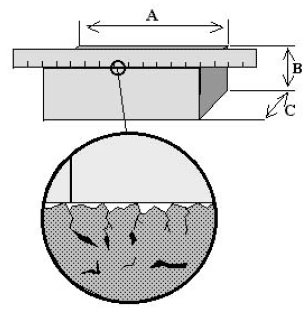Figure 1. A straightedge placed along the edge of a brick demonstrates the concept of ‘external volume,’ the volume contained by virtue of surface irregularities.

When a solid material is in granular or powdered form, the bulk contains another type of void: interparticle space. The total volume of interparticle voids depends on the size and shape of the individual particles and how well the particles are packed.

Table 1 provides ‘standard’ definitions for volume in consideration of these elemental volumes. Figure 2 illustrates the bases for the differences between various volume definitions.

Table 1. Definitions of various types of volumes. BSI = British Standards Institute, ASTM = American Society for Testing and Materials.

 Solid Material Volume Open Pore Volume Closed Pore Volume Inter - particle Void Volume External Void Volume Absolute powder volume: (also called Absolute volume): The volume of the solid matter after exclusion of all the spaces (pores and voids) (BSI). X Apparent particle volume: The total volume of the particle, excluding open pores, but including closed pores (BSI). X X Apparent powder volume: The total volume of solid matter, open pores and closed pores and interstices (BSI). X X X X Bulk volume: The volumes of the solids in each piece, the voids within the pieces, and the voids among the pieces of the particular collection (implied by ASTM D3766). X X X X X Envelope volume: The external volume of a particle, powder, or monolith such as would be obtained by tightly shrinking a film to contain it (BSI). The sum of the volumes of the solid in each piece and the voids within each piece, that is, within close-fitting imaginary envelopes completely surrounding each piece (Implied by ASTM D3766; see Table 2). X           X X           X X           X X X           X Geometric volume: The volumes of a material calculated from measurements of its physical dimensions. X X X X X Skeletal volume: The sum of the volumes of the solid material and closed (or blind) pores within the pieces (Implied by ASTM D3766). X X True volume: Volume excluding open and closed pores (implied by BSI). X Void: Space between particles in a bed (BSI). X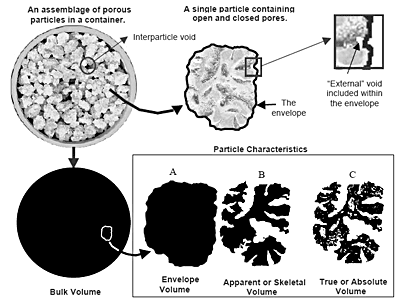Figure 2. Illustration of various volume types. At the top left is a container of individual particles illustrating the characteristics of bulk volume in which interparticle and “external” voids are included. At the top right is a single porous particle from the bulk. The particle cross-section is shown surrounded by an enveloping band. In the illustrations at the bottom, black areas shown are analogous to volume. The three illustrations at the right represent the particle. Illustration A is the volume within the envelope, B is the same volume minus the “external” volume and volume of open pores, and C is the volume within the envelope minus both open and closed pores.

Three volume definitions, those of apparent powder volume, bulk volume and envelope volume, have subtle differences. Apparent powder volume is most rigidly defined. It is the sum total of the four volumes indicated by the column headings in Table 1. Bulk and tap densities are obtained from bulk and tap volumes, which are apparent powder volumes obtained under specified conditions. Usually, this involves placing the powder into a rigid container of specific dimensions while taking specific steps to control compaction. In the case of a monolithic sample, bulk volume may be calculated from dimensional measurements or by displacement of some medium in which it is immersed.

## Difference Between Envelope and Bulk Volumes

The difference between envelope and bulk volumes often is unclear. As can be seen in Table 1, ASTM’s definition of envelope volume must be inferred from their definition of envelope density in Table 2. It implies that the definition pertains only to a single particle, while BSI’s definition encompasses a particle or a monolith (singular implied), and a powder (by definition, a collection of fine particles).

Table 2. Definitions of various types of densities that follow from the volume definitions of Table 1. BSI = British Standards Institute, ASTM = American Society for Testing and Materials.

 Solid Material Volume Open Pore Volume Closed Pore Volume Inter - particle Void Volume External Void Volume Absolute powder density: The mass of powder per unit of absolute volume (BSI). X Apparent particle density: The mass of a particle divided by its apparent (particle) volume (BSI). X X Apparent powder density: The mass of a powder divided by its apparent volume (BSI). X X X X Bulk density: (also called Bulk powder density): The apparent powder density under defined conditions (BSI). The mass of the particles divided by the volume they occupy that includes the space between the particles (ASTM D5004). The ratio of the mass of a collection of discrete pieces of solid material to the sum of the volumes of: the solids in each piece, the voids within the pieces, and the voids among the pieces of the particular collection (ASTM D3766). X             X X             X X             X X             X Envelope volume: The external volume of a particle, powder, or monolith such as would be obtained by tightly shrinking a film to contain it (BSI). The sum of the volumes of the solid in each piece and the voids within each piece, that is, within close-fitting imaginary envelopes completely surrounding each piece (Implied by ASTM D3766; see Table 2). X           X X           X X           X X           X Effective particle density: The mass of a particle divided by its volume including open pores and closed pores (BSI). X X X Envelope density: The ratio of the mass of a particle to the sum of the volumes of: the solid in each piece and the voids within each piece, that is, within close-fitting imaginary envelopes completely surrounding each piece (ASTM D3766). The ratio of the mass of a particle to the envelope volume of the particle (implied by BSI). X           X X           X X           X X X           X Skeletal density: The ratio of the mass of discrete pieces of solid material to the sum of the volumes of: the solid material in the pieces and closed (or blind) pores within the pieces (ASTM D3766). X X Tap density (also called Tap powder density): The apparent powder density obtained under stated conditions of tapping (BSI). X X X X Theoretical density: The ratio of the mass of a collection of discrete pieces of solid material to the sum of the volumes of said pieces, the solid material having an ideal regular arrangement at the atomic level (ASTM). X True density (also called True particle density); The mass of a particle divided by its volume, excluding open pores and closed pores (BSI). X

In regard to this document and others by Micromeritics, envelope volume and envelope density are defined following ASTM’s definition, that is, in terms of a single particle or monolith. Bulk properties pertain to collections of particles. A third definition, that of geometrical volume, is adopted and pertains to a volume calculated from the linear dimensions of the bulk or monolithic material.

## Specific Gravity

Specific gravity, in general, is the ratio of the weight in air of a given volume of material at a stated temperature to the weight of the same volume of water (or other reference) at a stated temperature. It, therefore, is dimensionless and is sometimes expressed in the form, for example, 6.25 25/25 C. In this format, 6.25 is the specific gravity value and 25/25 °C indicates that the sample temperature was 25 ° and the reference water temperature was 25 °C. The type volume measurement used in calculating density determines the type specific gravity. For example, true specific gravity is calculated using true volume measurements. It is the ratio of the true density of the material (determined at a specific temperature) to the true density of water (at a specific temperature).

The reason for expressing the temperatures is that the density of pure, air-free water at 3.98 C is 1.00000 g/ml. This is the maximum density value; density decreases with both higher and lower temperatures. If one assumes a density of 1.000 for water at room temperature the error introduced is about 0.3%.

## Using Manual Laboratory Devices

Although not a complete list, the following represents the most common methods by which volume and density are determined manually.

## Pycnometry (Specific Gravity Bottles)

A pycnometer is a vessel with a precisely known volume. When one thinks of density determinations, one usually thinks of a pycnometer. Although a pycnometer is used to determine density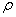or specific gravity, it measures volume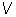; a balance is used to determine mass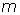. Manual pycnometers (glassware) typically are used to determine the density or specific gravity of liquids by filling the vessel, then weighing. Density is calculated by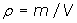and specific gravity by the same equation and dividing both sides by the density of water with reference to temperature.

Essentially the same process can be used to determine the volume of an unknown, enclosed space. First the object containing the void is weighed empty. It is then filled with a liquid of known density and reweighed. The weight difference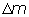is the weight of the liquid and from these data, volume can be calculated by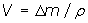As will be explained, this process is used to ‘calibrate’ sample cells used in mercury porosimetry.

Another pycnometer method is to place a quantity of a dry, pre-weighed solid sample in the pycnometer and fill the rest of the pycnometer with a liquid of known density (typically water), the weight of the pycnometer filled only with the liquid having previously been established. The density of the sample can be determined from the known density of the water, the weight of the pycnometer filled only with the liquid, the weight of the pycnometer containing both sample and liquid, and the weight of the sample. This is a common method used in characterizing soil samples.

## Hydrostatic Weighing (Displacement Method)

By this method, the volume of a solid sample is determined by comparing the weight of the sample in air to the weight of the sample immersed in a liquid of known density. The volume of the sample is equal to the difference in the two weights divided by the density of the liquid.

Conversely, if the volume of a solid object is accurately known, the density of the liquid can be determined by the loss of weight of the immersed object. This is the basis for the hydrometer method (see next section).

If the sample is porous, one must determine if the pores are to be included or excluded from the volume. If they are to be included or the sample will react with the displacement medium, a sealing coating can be applied (see Bulk / Envelope Volume by Coating). If pore volume is to be excluded, the liquid must displace the air and completely fill the pores.

Various pretreatment methods are used including evacuation and boiling.

When determining volume by directly measuring the displaced volume, liquids, fine particles or gases can be used as the displacement medium. If the sample material is porous, fine particles will not penetrate into the smaller pores that water can enter. Mercury, being a non-wetting liquid, also will not penetrate pores under ambient pressure as will wetting liquids. Gases, Helium in particular, will penetrate readily into very fine pores.

## Hydrometers

A hydrometer is a vertical float that measures the density or specific gravity of a liquid or liquid/solid suspension (slurry). The hydrometer, inscribed with a graduated scale along its length, sinks into the liquid until it has displaced a volume of liquid equal in weight to that of the float. Specific gravity or density is read directly from the inscribed scale at the liquid surface after buoyancy and gravitational forces equalize.

## Float-Sink or Suspension (Buoyancy) Method

This method requires a liquid of known and adjustable density in which the sample is placed. The density of the liquid is adjusted until the sample either begins to sink or float, or is suspended at neutral density in the liquid.

The density of the object is then equated to that of the liquid. This method also is used to separate materials by their density.

A density gradient column is a column of liquid that varies in density with height. A sample is placed in the liquid and observed to determine at what vertical level in the column the sample is suspended. The density of the liquid at that level is the density of the sample, and that value is determined by standards of known density.

## Tap Density and Vibratory Packing Density

These are very similar methods for determining the bulk density of a collection of particles under specific conditions of packing. In the former case, packing is achieved by tapping the container and in the latter by vibrating the container. The particles under test should not break up under test conditions.

## Bulk / Envelope Volume by Coating

Coating the sample allows determination of bulk volume or apparent volume of solids while preventing absorption or reaction with suspension liquids.

Penetration of the coating into the open pores of the sample must be considered.

Following the referenced method, the mass of the sample is obtained. The sample is dipped into molten wax of known density. After withdrawal, any air bubbles in the wax coating are pressed out, and the coated sample is weighed. The difference in weight before and after coating is the weight of the wax, and dividing this number by the density of the wax provides the volume of wax composing the coating. The volume of the coated sample is determined by hydrostatic weighing. From this volume, the volume of wax (or other coating) is subtracted, yielding the bulk (or envelope) volume of the sample.

## Volume and Density Determinations by Laboratory Analytical Instruments

The displacement method is the underlying principle used in all automated volume determining methods discussed below.

## Skeletal Volume and Density by Gas Pycnometry

A gas pycnometer operates by detecting the pressure change resulting from displacement of gas by a solid object. Figure 3 helps explain the technique. An object of unknown volume Vx is placed into a sealed sample changer of known volume Vs. After sealing, the pressure within the sample chamber is measured Ps. Then, an isolated reference chamber of known volume Vr is charged to a pressure Pr, which is greater than that of the sample chamber. A value isolating the two chambers is opened and the pressure Psys of the system is allowed to equilibrate. The gas law, PV = nRT is applied to determine the volume of the unknown as follows:

Assume the system is maintained at a constant temperature T and there is no net loss or gain of gas, that is, the number of gas molecules n is constant throughout the experiment.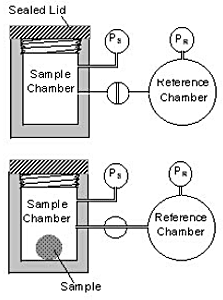Figure 3. Essentials of the operation of a precalibrated gas pycnometer.

Logically, one deduces that when the valve is opened the pressure in the reference volume will fall and the pressure in the sample chamber will rise. The larger the volume of the unknown, the higher will be the final system pressure, the initial pressure of the reference chamber being the upper limit when 100 percent of the volume of the sample chamber is displaced by the unknown volume.

Mathematically, the initial condition is

Ps(Vs – Vx) + PrVr = nRT

where R is the gas constant.

After the valve is opened, the condition changes to

Psys(Vs + Vr – VX) = nRT

Ps(Vs – Vx) + PrVr = Psys(Vs + Vr – VX)

which can be solved in terms of the unknown

quantity Vx yielding,

VX = (PsysVs + PsysVr – PsVs – PrVr) / (Psys-Ps)

The accuracy and precision of the gas pycnometer in the determination of skeletal volume and density can be quite high, but relies greatly on the sample material and analysis gas being free of moisture. The sample also must be free of any volatile substances that can contribute their partial pressures and cause error and instability. For these reasons, the gas is a pure gas or dry air, and the sample is pretreated in a vacuum oven to remove volatiles. The contribution of the instrument to error is, for the most part, confined to leaks and temperature instability or temperature gradients.

Helium typically is the gas used because it readily diffuses into small pores. Other gases also are used and selected based on the size of the molecule or the way in which the gas reacts with the surface of the unknown sample.

Sometimes, the difference in results obtained when using different gases is indicative of some sought-after characteristic of the sample.

The gas pycnometer is used in a wide variety of applications and found in a number of configurations - manual and automated, single sample chamber and multi-chambered, and fixed chamber volume and multiple volume designs.

One variation of the design makes it especially suitable for the measurement of rigid, closed cell foams. The applications of this technique are not only for material volume and density, but also as a means for porosity determination as discussed in a later section. Citations to several diverse applications are found in the reference section, the materials under study being pitch, coatings, petroleum coke , cereal grain, tuff cores, volcanic soils, wool, compost, asteroids, chromatographic packing materials and cellulose powder.

## Envelope Volume and Density by Displacement of a Dry Medium

The displacement technique applies to a solid object immersed in a bed of much smaller solid particles as well as in liquids and gases. The difference is in the way the displaced medium conforms to the surface of the immersed object.

A liquid can conform quite closely to the surface. Wetting liquids have the capability to fill voids and pores that communicate with the surface. Solid particles and non-wetting liquid displacement media do not invade pores and provide means by which envelope density can be determined in a controlled manner. The use of a non-wetting liquid (mercury, specifically) is discussed in the next section.

## Micromeritics GeoPyc Model 1360

Micromeritics’ GeoPyc Model 1360 is the only known-of commercial instrument that automatically determines the volume and density of a solid object by displacement of a solid medium. The medium is a narrow distribution of small, rigid spheres that have a high degree of flowability and achieve close packing around the object under investigation.

The particles are sufficiently small that during consolidation they conform closely to the surface of the object, yet do not invade pore space.

Repeatability and reproducibility are achieved by a controlled method of compaction. The sample cell in which the dry medium is placed is a precision cylinder. A plunger compresses the powder as the cell vibrates; the force of compression is selectable and, therefore, repeatable from test to test. A preliminary compaction with only the displacement medium in the cell establishes a zero-volume baseline.

The object is then placed in the cylinder with the dry medium and the compaction process is repeated. The difference in the distance ht the piston penetrates the cylinder during the test and the distance h0 it penetrates during the baseline procedure (h = h0 – ht) is used to calculate the displacement volume of the medium using the formula for the volume of a cylinder of height h.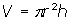Figure 4 illustrates the process. This relatively new technique is finding applications where tap density and mercury displacement methods traditionally have been used.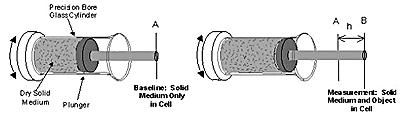Figure 4. Volume determination by the displacement of a dry medium.

## Bulk, Envelope, and Skeletal Volumes and Densities by Mercury Porosimetry

Mercury is a non-wetting liquid that must be forced to enter a pore by application of external pressure. The surface tension of mercury and the interfacial tension between mercury and the solid surface results in mercury bridging the openings to pores, cracks, and crevices until sufficient pressure is applied to force entry.

For example, at atmospheric pressure, mercury will resist entering pores smaller than about 6 micrometers in diameter. When an object is surrounded by mercury, the mercury forms a closely fitting liquid envelope around the object. How closely the mercury conforms to the surface features of the object depends on the pressure applied. At some pressure, mercury begins to enter the pores, cracks, crevices, and voids of the sample. At a pressure of 60,000 psi (414 MPa) mercury has been forced to enter pores of diameters down to 0.003 micrometer. This fills essentially all pore volume in most materials.

There is a slight but important difference in the method of determining the volume of a solid object and that of a finely divided powder by mercury porosimetry. Therefore, the two forms of sample materials are considered separately in the subsequent discussion.

## Monolithic Sample Material

First, consider a single lump of solid material of known mass. It is assumed that the exact volume of the sample cell has been established using the method described in a previous section on manual pycnometry. The sample cell (referred to as a penetrometer or dilatometer) containing the sample is evacuated and filled with mercury. Mercury surrounds the sample, but, at sub-ambient or near-ambient pressure, does not enter small cracks and crevices in the surface nor into pores in the structure of the material. Reweighing the filled sample containers and subtracting from this the weight of the empty sample cell plus sample, yields the weight of the surrounding mercury from which the volume of mercury is to be calculated. The difference in the volume of the empty sample cell and the calculated volume of mercury is equal to the envelope volume of the sample.

## Skeletal Volume

The skeletal volume of the sample also can be determined by increasing pressure and causing the mercury to invade the open pore space. If, at maximum pressure, all open pores in the sample are filled, then the volume of mercury intruded is equal to total pore volume. This value subtracted from the bulk or envelope volume of the monolithic sample yields its skeletal volume.

If the sample contains no closed (blind) pores, then the volume measured is the true volume.

Finely grinding materials with closed pores (when appropriate) may allow true volume to be determined by making these pores accessible to the surface.

If the sample contains pores smaller than the minimum pore size into which mercury can intrude at maximum instrument pressure, then the accuracy of skeletal volume determination is affected. For these samples, skeletal volumes would be less than those obtained determined by gas pycnometry because gases such as helium and nitrogen can penetrate into micropores and small mesopores where mercury cannot. The difference in skeletal volume obtained by mercury porosimetry and that obtained by gas pycnometry indicates pore volume in the size range from the minimum size probed by mercury porosimetry down to approximately the size of the gas molecule.

## Powdered or Granulated Sample Materials

In the second case where the sample material is a fine powder or granules, the procedure follows essentially the same preliminary steps as when the sample is a single piece. The difference is that there is an additional step in the interpretation and reduction of the experimental data.

A powdered sample is a bulk mass of grains; at low pressure mercury will not invade the interparticle voids. This is illustrated by Illustration A in Figure 5.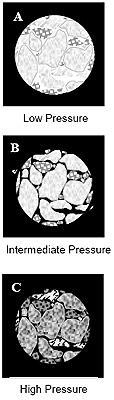Figure 5. Mercury intrusion into pore space as pressure increases; black areas indicate mercury. A. Mercury envelops the mass. B. Mercury fills the interparticle voids. C. Mercury penetrates into the pores of the individual particles.

Initially, the mercury envelope forms around the bulk mass and not around the individual particles, so the bulk volume or envelope volume (according to the definition adopted) of the entire sample mass is displaced. Only when pressure is increased will mercury invade the interparticle space and envelope individual particles (Illustration B, Figure 5). At what pressure interparticle void filling begins (the breakthrough pressure) and the pressure at which it is completed depends on the size and shape of the particles and can be readily identified on a plot of volume intruded versus applied pressure. The indication of breakthrough is an abrupt increase in the slope of the intrusion curve and, when filling is completed, a notable decrease.

A further increase in pressure will force mercury into the voids within the individual particles (Illustration C, Figure 5). Only pores with access to the surface can be filled and any blind pores remain unfilled. Further increases in pressure can cause temporary or permanent structural changes in the sample material.

The critical points during the mercury intrusion process are illustrated in Figure 6.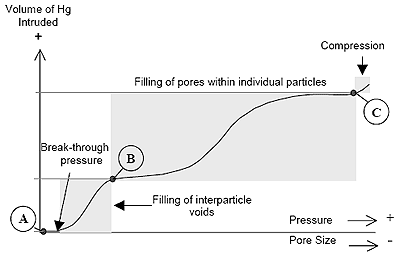Figure 6. The intrusion volume points on a mercury intrusion plot that are critical in the determination of volume and density. Point A is used to determine bulk or envelope volume, points A and B are used to determine interparticle void volume, and points A and C are used to determine skeletal volume.

When measuring volume (density) by mercury porosimeter, it should be recognized that the value obtained is pressure-dependent (porefilling dependent). Since the mercury porosimeter provides a continuous record of mercury volume change within the sample cell, the volume and density at any pressure can be determined. Typically, the volume of mercury displaced at minimum pressure and that displaced at maximum pressure (prior to deformation) are used to determine bulk (or envelope) density and skeletal density, respectively. For powders, an intermediate volume, the total volume of the grains only, may be determined.

A mercury porosimeter is seldom used solely for the determination of envelope, bulk, and skeletal volume determinations. These determinations more often are a by product of a data set that was obtained primarily for the determination of pore volume distribution by pore size.

## Porosity Information Derived from Volume and Density Determinations

The subject of porosity was touched upon in the individual sections above as it relates to determining material volume. Material porosity is approached in this section as the primary physical characteristic of interest. However, only the analytical methods and techniques used to determine material volume are considered, so, by these analytical methods, porosity information is a byproduct of volume determinations and not the primary emphasis.

Table 3 contains various definitions of porosity. These are only a few examples and the same term may have slightly different meanings in different applications. For example, ASTM defines the term ‘porosity’ in over a dozen different ways.

Table 3. Porosity terms adapted from various sources including British Standards Institution, International Union of Pure and Applied Chemistry, American Society for Testing and Materials, and U.S. GeologicalSurvey.

 Porosity Term Definition Interstice / Interstitial Void An opening in a rock or soil that is not occupied by solid matter (USGS) Void space between particles Macropore A pore of diameter greater than about 50nm Mesopore A pore of diameterfrom about 2nm to 50nm Micropore A pore of diameter less than about 2nm Pore diameter The diameter of a pore in a model in which the pores typically are assumed to be cylindrical in shape and which is calculated from data obtained by a specified procedure Pore volume, specific Pore volume per unit mass of material Pore volume The volume of open pores unless otherwise stated Pore, closed A cavity with no access to an external surface Pore, ink-bottle An open pore with a narrow neck Pore, open A cavity or channel with access to an external surface Porosity, effective The ratio, usually expressed as a percentage of the total volume of voids available for fluid transmission to the total volume of the porous medium Porosity, interparticle Void space between particles Porosity, intraparticle All porosity within the envelopes of individual particles Porosity, particle The ratio of the volume of open pore to the total volume of the particle Porosity, powder The ratio of the volume of voids plus the volume of open pores to the total volume occupied by the powder Porosity (a)The ratio of open pores and voids to the envelope volume (BSI) (b) The ratio, usually expressed as a percentage, of the total volume of voids of a given porous medium to the total volume of the porous medium (ASTM) Void The space between particles in a bed

Depending upon the measurement method, various types of volumes as defined in Table 1 can be determined. Obtaining two or more volume values by different methods allows extraction of porosity information by the application of simultaneous equations. The set of equations implied by Table 1 is:

## Bulk Volume

VB = VS + VOP + VCP + VI + VExt

## Apparent Particle Volume

VAPart = VS + VCP

## Apparent Powder Volume

VAPow = VS + VOP + VCP + VI

## Envelope Volume (BSI)

VE = VS + VOP + VCP + VI + VExt

## Envelope Volume (ASTM)

VE = VS + VOP + VCP + VExt

VSk = VS + VCP

## True Volume

VT = VS

In the above equations, VS is the volume of the solid material, VOP the volume of open pores, VCP the volume of closed pores, VI the volume of interparticle voids, and VExt the external void volume. Any of these equations can be rearranged and solved for pore volume. Examples follow.

## Percent Porosity

From measurements of bulk volume (VB) and skeletal volume (VSk), total porosity VPt can be determined from the equation VPt = VB - VSk. This allows percent porosity to be calculated by the simple relationship

% Porosity =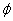= (VPt/VB) x 100%.

## Percent Porosity Filled

A mercury porosimeter tracks the volume of mercury intruded VI into the sample from minimum to maximum pressure. Since the total volume of mercury injected into the sample equals the total volume (VPt) of open pores, the percent of pore volume filled at any pressure can be determined by

%VP Filled = (VI / VPt) x 100%.

If the sample contains closed pores, then the above equation becomes

% VP Filled = ((VI + VPc) / VPt) x 100%.

The size of pores being invaded by mercury depends on the pressure applied. This means that, at a specific pressure (pore size), the percent porosity filled relates to pores of the current size and larger. The remaining percent of unfilled pores relates only to pores smaller than the current size.

## Apparent (Skeletal) Volume, Bulk Volume and Open Porosity by Liquid Absorption

To determine the volume of open pores in a sample, first the mass of the dry sample is obtained. Then, the sample is immersed in a liquid that is capable of penetrating into the open voids. When u sing water, boiling may be required to assure pore filling. Skeletal volume is determined by hydrostatic weighing in the same liquid. The sample is weighed again after removing it from the liquid. The difference between the wet and dry mass divided by the density of the liquid is the volume of open pores in which the liquid washable to penetrate.

A variation of this method uses oil as the liquid. To assure adequate pore filling, the sample is immersed in oil and the container evacuated to a few mmHg and maintained for 1 hour. Atmospheric pressure is restored and the sample is left to equilibrate for 30 minutes.

## True Volume and Closed Pore Volume by Size Reduction

Methods of determining bulk volume and open pore volume have been described. However, material may contain closed pores. If the true density of the solid material is known, then the mass of the sample divided by its density is its true volume; bulk volume minus open pore volume minus true volume is the volume of closed pores.

If the density of the solid material is not known, but its bulk volume and open pore volume have been determined, the volume of closed pores may be found by grinding the sample into a powder. Any remaining closed pores will be smaller than the particle size of the powder. The true volume of the sample (the powder) is determined by liquid or gas displacement.

## Total Pore Volume

Bulk volume and true volume having been obtained for a sample by one of the methods above, the difference between the former and latter is total pore volume. Likewise, if open pore volume and closed pore volume are determined as suggested above, their sum is total pore volume.

## Conclusions

Density, volume, and porosity are physical characteristics of solid materials that can be determined by a variety of experimental techniques. However, the value obtained is very likely to be dependent on the technique. This is largely because of the way the measurement technique treats volume in respect to the degree of exclusion of void spaces associated with the sample material. Various definitions of density and volume are used to differentiate these values in terms of what void volumes are included with the overall volume determination. An analyst must understand the type of volume or density sought in order to select the appropriate measurement technique.This information has been sourced, reviewed and adapted from materials provided by Micromeritics Instrument Corporation.

## Citations

• APA

Micromeritics Instrument Corporation. (2019, December 09). Volume and Density Definitions and Determination Methods. AZoM. Retrieved on September 18, 2020 from https://www.azom.com/article.aspx?ArticleID=3214.

• MLA

Micromeritics Instrument Corporation. "Volume and Density Definitions and Determination Methods". AZoM. 18 September 2020. <https://www.azom.com/article.aspx?ArticleID=3214>.

• Chicago

Micromeritics Instrument Corporation. "Volume and Density Definitions and Determination Methods". AZoM. https://www.azom.com/article.aspx?ArticleID=3214. (accessed September 18, 2020).

• Harvard

Micromeritics Instrument Corporation. 2019. Volume and Density Definitions and Determination Methods. AZoM, viewed 18 September 2020, https://www.azom.com/article.aspx?ArticleID=3214.